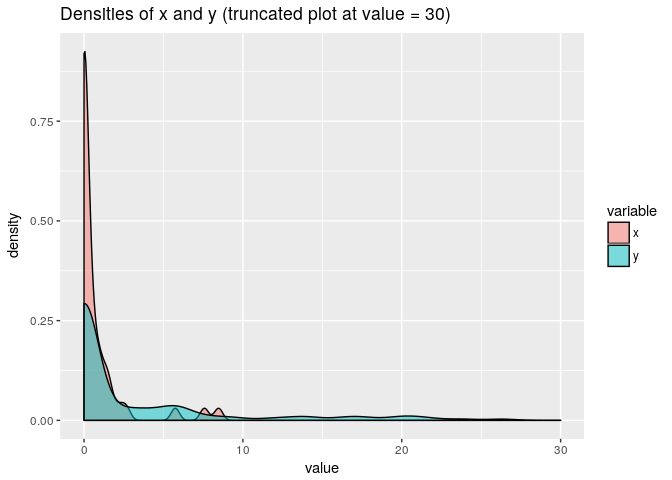# rbtt (Robust bootstrap-based t-test)

## Overview

rbtt is an alternative bootstrap-based t-test aiming to reduce type-I error for non-negative, zero-inflated data

Tu & Zhou (1999) showed that comparing the means of populations whose data-generating distributions are non-negative with excess zero observations is a problem of great importance in the analysis of medical cost data. In the same study, Tu & Zhou discuss that it can be difficult to control type-I error rates of general-purpose statistical tests for comparing the means of these particular data sets. This package allows users to perform a modified bootstrap-based t-test that aims to better control type-I error rates in these situations.

## Usage

Let’s say we have some non-negative data with clumping at zero:

``````x <- rbinom(50, 1, 0.5) * rlnorm(50, 0, 1)
y <- rbinom(150, 1, 0.3) * rlnorm(150, 2, 1)
``````Then we may compute rbtt-based t-tests to compare the means:

``````# Use ‘method = 1’ for a two-sample, two-sided rbtt under the equal variance assumption,
rbtt(x, y, n.boot=999, method = 1)

##
##  Two-sided robust bootstrapped t-test assuming equal variance
##
## data:  x and y
## t = -2.3, p-value = 0.03
## alternative hypothesis: true difference in means is not equal to 0
## 95 percent confidence interval:
##  -7.2644 -0.1393
## sample estimates:
## mean of x mean of y
##    0.8163    5.1450

# Use ’method = 2' for a two-sample, one-sided rbtt without the equal variance assumption
rbtt(x, y, n.boot=999, method = 2)

##
##  One-sided robust bootstrapped t-test not assuming equal variance
##
## data:  x and y
## t = -4, p-value <2e-16
## alternative hypothesis: true difference in means is less than 0
## 95 percent confidence interval:
##  -6.932 -1.726
## sample estimates:
## mean of x mean of y
##    0.8163    5.1450
``````

Alternatively, you can specify `method = "both"` to perform both methods simultaneously (this is also done by default).

## Parallelize rbtt

``````# Compare speed when using single-core versus multiple-core rbtt on 99999 bootstrap resamples
system.time(rbtt(x, y, n.boot = 99999, method = 1, n.cores = 1))

##    user  system elapsed
##   8.150   0.011   8.198

system.time(rbtt(x, y, n.boot = 99999, method = 1, n.cores = 3))

##    user  system elapsed
##   6.617   0.078   3.782
``````

## Comparison between rbtt and t.test

First, we perform some simulations.

``````n.sim <- 999

t.test.results <- numeric(n.sim)
rbtt.results <- numeric(n.sim)

pval.table.list <- mclapply(1:n.sim, function(i)
{
# True means are equal
x <- rbinom(50, 1, 0.5) * rlnorm(50, 1.15, 1)
y <- rbinom(150, 1, 0.5) * rlnorm(150, 1.15, 1)

t.test.result <- t.test(x, y)\$p.value
rbtt.result <- rbtt(x, y, n.boot = 999, method = 1)\$p.value

return(c(t.test.result, rbtt.result))
}, mc.cores = 4)

pval.table <- do.call(rbind, pval.table.list)
``````

Now, let’s evaluate the type-I error of these simulations using a significance level of 0.05.

``````# t.test type-I error with significance level of 0.05:
sum(pval.table[,1] < 0.05) / n.sim

##  0.06006

# rbtt type-I error with significance level of 0.05:
sum(pval.table[,2] < 0.05) / n.sim

##  0.05005
``````

More accurate p-values and type-I error estimates can be obtained by increasing `n.boot` and `n.sim`, respectively

## Contributors

• Ian Waudby-Smith (University of Waterloo)

• Dr. Pengfei Li (University of Waterloo)